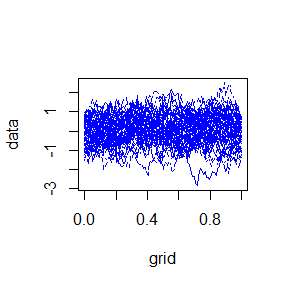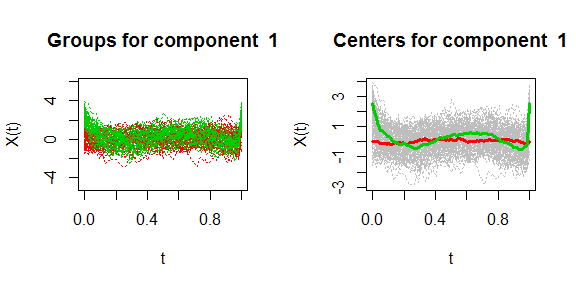# Introduction

Package gmfd (Generalized Mahalanobis Functional Distance) is an R package which gathers statistical methods for both inference and clustering of functional data based on a generalization of Mahalanobis distance. The package supports both univariate and multivariate functional data.

# Simulation of functional data

Let us consider a process $$X(t) \in L^2(I)$$, with $$I \in \mathbb{R}$$, with mean function $$m(t) = \mathbb{E}[X(t)]$$ and covariance operator $$V$$, i.e. V is a linear compact integral operator from $$L^2(I)$$ to $$L^2(I)$$ such that $$(Va)(s) = \int_I v(s,t) a(t) dt \quad \forall a \in L^2(I)$$, where $$v$$ is the covariance function defined as $$v = \mathbb{E}[(X(t) - m(t))(X(s)-m(s))]$$. Then, denote with $$\{\rho_k, k \ge 1\}$$ and $$\{\theta_k, k \ge 1\}$$ respectively the sequences of eigenvalues and eigenfunctions of $$v$$. The Karhunen-Loève expansion decomposes the process $$X(t)$$ in a sum of its mean $$m(t)$$ and the series of orthonormal functions $$\theta_k(t)$$, each one multiplied by zero-mean uncorrelated random variables $$\sqrt{\rho_k} Z_k$$, ($$\rho_k>0, \mathbb{V}ar(Z_k) = 1)$$. Then we can write $\begin{equation*} X(t) = m(t) + \sum_{k=1}^\infty Z_{k} \sqrt{\rho_k} \cdot \theta_k(t) \end{equation*}$

The function gmfd_simulate( size, mean, covariance, rho, theta ) can simulate an univariate sample of functional data where size represents the number of elements to be generated while mean is the center of the distribution. The user can choose to use the argument covariance for the covariance of the data or alternatively the sequences of eigenvalues and eigenfunctions of the covariance matrix.

library( gmfd )

# Define the parameters
n <- 50
P <- 100
K <- 150

# Grid of the functional dataset
t <- seq( 0, 1, length.out = P )

# Define the means and the parameters to use in the simulation
m1 <- t^2 * ( 1 - t )

rho <- rep( 0, K )
theta <- matrix( 0, K, P )
for ( k in 1:K ) {
rho[k] <- 1 / ( k + 1 )^2
if ( k%%2 == 0 )
theta[k, ] <- sqrt( 2 ) * sin( k * pi * t )
else if ( k%%2 != 0 && k != 1 )
theta[k, ] <- sqrt( 2 ) * cos( ( k - 1 ) * pi * t )
else
theta[k, ] <- rep( 1, P )
}

# Simulate the functional data
X <- gmfd_simulate( size = n, mean = m1, rho = rho, theta = theta )

# S3 class funData for functional data

For ease of manipulation and visualization, a S3 class for both univariate and multivariate functional data has been created. A funData object represents a functional data and it is defined by the function funData( grid, F ) where grid is the grid of evenly spaced points over which the functional data is defined and F is a matrix (if it is a univariate functional data) or a list (if it is a multivariate functional data). A functional data as it has been just described can be then represented by using the function plot.funData which takes as argument all the usual customisable graphical parameters.

# Create a functional data object
FD1 <- funData( t, X )

# Plot the funData object
plot( FD1, col = "blue" , xlab = "grid", ylab = "data")# Generalized Mahalanobis Distance

Let us consider a $$J$$-dimensional sample of $$n$$ realizations $$\mathbf{X}_1(t), ...,\mathbf{X}_n(t)$$ of a stochastic process in $$(L^2(I))^J$$, with $$J\geq 1$$. Let $$\bar{\mathbf{X}}_n(t) = n^{-1}(\mathbf{X}_1(t) + \ldots + \mathbf{X}_n(t))$$ be the empirical mean. The estimated covariance function is defined as follows: $\begin{equation} \label{hat_v} \hat{v}(s,t) := \frac{1}{n-1} \sum_{i=1}^n \big(\mathbf{X}_i(s) - \bar{\mathbf{X}}_n(s)\big)\big(\mathbf{X}_i(t) - \bar{\mathbf{X}}_n(t)\big)^\top, \end{equation}$ from which we can compute the sequences of its eigenfunctions $$\{\hat{\boldsymbol{\varphi}}_{k}=(\hat{\varphi}_k^{(1)},..., \hat{\varphi}_k^{(J)})^\top,\, k\geq 1\}$$ and the associated eigenvalues $$\{\hat{\lambda}_k;\, k \geq 1\}$$. Since in this case the covariance function is computed using $$n$$ curves, we have $$\hat{\lambda}_k = 0$$ for all $$k\geq n$$, and hence the functions $$\{\hat{\boldsymbol{\varphi}}_k;\, k \geq n\}$$ can be arbitrary chosen such that $$\{\hat{\boldsymbol{\varphi}}_k;\, k \geq 1\}$$ is an orthonormal basis of $$(L^2(I))^J$$. The empirical version of the generalized Mahalanobis distance based on the covariance estimator $$\hat{v}$$ can be written as follows: \begin{equation}\begin{aligned} \label{def:hat_dp} \hat{d}^2_p(\textbf{X}_i(t),\textbf{X}_j(t)) &= \sum_{k=1}^{\text{min}\{n-1,P\}} \hat{d}^2_{M,k}(\textbf{X}_i(t), \textbf{X}_j(t)) \hat{h}_k(p) \\ & + \sum_{k=\text{min}\{n-1,P\}+1}^{P} p \Big( \langle \textbf{X}_i(t) - \textbf{X}_j(t), \hat{\boldsymbol{\varphi}}_k \rangle \Big)^2, \end{aligned} \end{equation}

where $$P$$ is the length of the independent variable grid, while $$\hat{d}^2_{M,k}(\cdot,\cdot)$$ and $$\hat{h}(p)$$ represent the estimates of the square of the contribution to this distance along the $$k$$-th component and the regularizing function, respectively. The function funDist( FD1, FD2, metric, p, lambda, phi ) computes the distance between two functional data FD1 and FD2 by using the chosen metric. The last three parameters are used only for the generalized Mahalanobis distance.

# We estimate the eigenvalues and eigenfunctions of the covariance matrix of data
lambda <- eigen( cov( FD1$data[] ) )$values
phi <- eigen( cov( FD1$data[] ) )$vectors

# Extract two curves from the samples to compute the distance between them
x <- funData( t, FD1$data[][1, ] ) y <- funData( t, FD1$data[][2, ] )

distance <- funDist( x, y, metric = "mahalanobis", p = 10^5, lambda, phi )
distance
##  2.306482

It is also possible to compute the dissimilarity matrix of a given sample by using the function gmfd_diss( FD, metric, p ).

# Inference on the means of functional data: two sample hypotesis tests

We want now to compare the means of two functional data samples. We simulate two samples $$\mathbf{X}_1(t), ...,\mathbf{X}_{n_1}(t)$$ and $$\mathbf{Y}_{n_2}(t), ...,\mathbf{Y}_{n_2}(t)$$ and consider the following asymptotic hypotesis test: $\begin{equation} H_0 : m_{1} = m_{2} \qquad \text{vs} \qquad H_1: m_{1} \neq m_{2}. \end{equation}$

where $$m_1$$ and $$m_2$$ are the real means of the two simulated samples. We can infer on the means of the two samples by using the function gmfd_test(FD1, FD2, conf.level, stat_test, p) where we have the two samples FD1 and FD2, the confidence level for the test conf.level, a string to choose the test statistic to use stat_test and the parameter of the regularizing function p. The function then returns the value of the test statistics, the value of the quantile and the p-value for the test.

# Simulate another functional sample
s <- 0
for ( k in 4:K ) {
s <- s + sqrt( rho[k] ) * theta[k, ]
}

m2 <- m1 + s
Y <- gmfd_simulate( n, m2, rho = rho, theta = theta )
FD2 <- funData( t, Y )

test_output <- gmfd_test(FD1, FD2, conf.level = 0.95, stat_test = "mahalanobis", p = 10^5)
test_output
## $T0 ##  74.00768 ## ##$quantile
##  12.52993
##
## $pval ##  0 # Clustering: the k-means algorithm The functional $$k$$-means clustering algorithm is an iterative procedure, alternating a step of cluster assignment, where all the curves are assigned to a cluster, and a step of centroid calculation, where a relevant functional representative (the centroid) for each cluster is identified. More precisely, the algorithm is initialized by fixing the number $$k$$ of clusters and by randomly selecting a set of $$k$$ initial centroids $$\{\boldsymbol{\chi}_1^{(0)}(t), \ldots , \boldsymbol{\chi}_k^{(0)}(t)\}$$ among the curves of the dataset. Given this initial choice, the algorithm iteratively repeats the two basic steps mentioned above. Formally, at the $$m^{th}$$ iteration of the algorithm, $$m\geq 1$$, the two following steps are performed: • Step 1 (cluster assignment step): each curve is assigned to the cluster with the nearest centroid at the $$(m-1)^{th}$$ iteration, according to the distance $$\hat{d}_p$$. Formally, the $$m^{th}$$ cluster assignment $$C_i^{(m)}$$ of the $$i^{th}$$ statistical unit, for $$i=1,\ldots,n$$, can be written as follows: $\begin{equation} C_i^{(m)} := \underset{l=1,\ldots,k}{\operatorname{argmin}}\:\hat{d}_p(\textbf{X}_i(t), \boldsymbol{\chi}_l^{(m-1)}(t)); \end{equation}$ • Step 2 (centroid calculation step): the computation of the centroids at the $$m^{th}$$ iteration is performed by solving the optimization problems: for any $$l=1,\ldots,k$$, $\begin{equation} \boldsymbol{\chi}_l^{(m)}(t) := \underset{\boldsymbol{\chi} \in (L^2(I))^J}{\operatorname{argmin}} \sum_{i:C_i^{(m)} = l} \hat{d}_p(\textbf{X}_i(t),\boldsymbol{\chi}(t))^2, \end{equation}$ where $$C_i^{(m)}$$ is the cluster assignment of the $$i^{th}$$ statistical unit at the $$m^{th}$$ iteration. The algorithm stops when the same cluster assignments are obtained at two subsequent iterations, i.e. the set of cluster assignments $$\{C_1^{(\bar{m})},\ldots,C_n^{(\bar{m})}\}$$ and the set of centroids $$\{\boldsymbol{\chi}_1^{(\bar{m})}(t),\ldots, \boldsymbol{\chi}_k^{(\bar{m})}(t)\}$$ are considered final solutions of the algorithm if $$\bar{m}$$ is the minimum integer such that $$C_i^{(\bar{m}+1)} \equiv C_i^{(\bar{m})}$$ for all $$i=1,\ldots,n$$. We apply the procedure by merging the two samples $$\mathbf{X}_1(t), ...,\mathbf{X}_{n_1}(t)$$ and $$\mathbf{Y}_{n_2}(t), ...,\mathbf{Y}_{n_2}(t)$$ previously simulated using the function gmfd_kmeans( FD, n.cl , metric, p ) where n.cl is the number of cluster. It returns a vector of the clusters and a vector or a list of vectors of the centers, other than a plot of the clusters along with their empirical means. # We estimate the eigenvalues and eigenfunctions of the covariance matrix of all merged data lambda <- eigen( cov( rbind( FD1$data[], FD2$data[] ) ) )$values
phi <- eigen( cov ( rbind( FD1$data[], FD2$data[] ) ) )\$vectors

# Functional data sample containing the merged samples
FD <- funData( t, rbind( X, Y ) )

kmeans_output <- gmfd_kmeans( FD, n.cl = 2, metric = "mahalanobis", p = 10^5 )